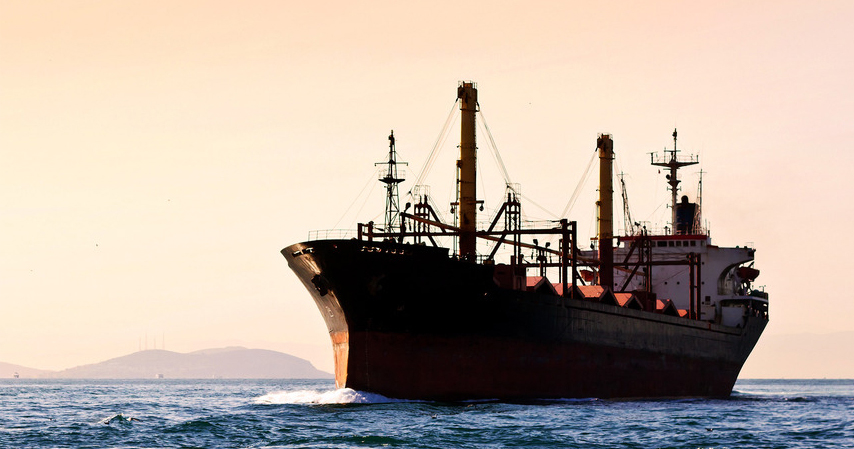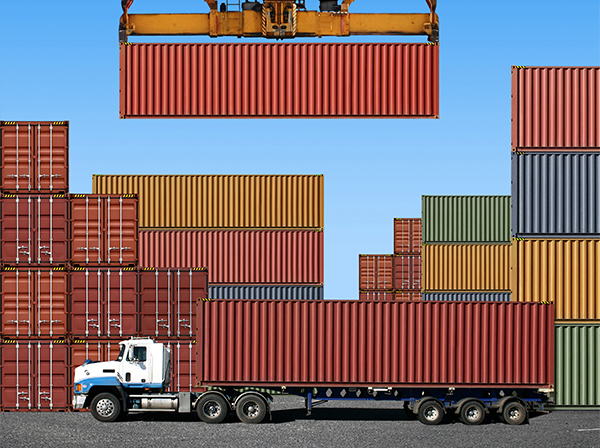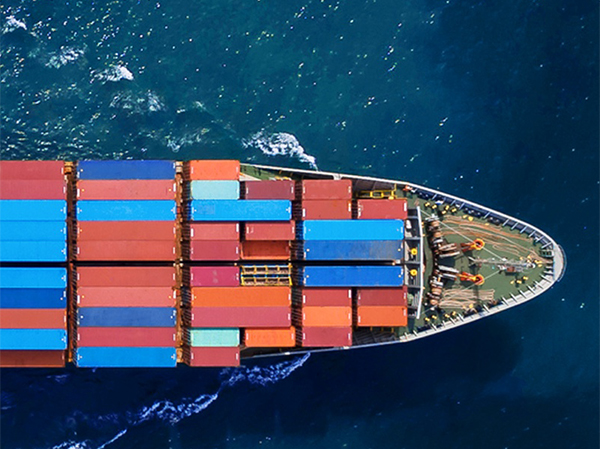Ocean Freight

CBM- Cubic meter

CFT- Cubic foot

THC- Terminal handling charges

FCL- Full container load (20 or 40 feet)

*LCL is based on cubic meters.( To calculate) L"xW"xH"/1728=Cubic feet
Cubic feet x .02832= Cubic meter•Ground Freight

B-train- 2-20ft. trailers being pulled by one tractor

CWT- Per hundred pounds

PUP- 20ft. trailer

Dimensional Freight- Subject to 10# per cubic ft.

• Calculation

Calculate Kilograms to Pounds

kgs x 2.2= pounds

Calculate Pounds to Kilograms

Pounds/2.2= kgs

Dimensional weight

L"xW"xH"/366= kgs
L"xW"xH"/166= pounds